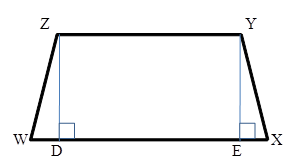Chapter 4.4, Problem 19EElementary Geometry For College St...

7th Edition
Alexander + 2 others
ISBN: 9781337614085

Solutions

Chapter
SectionElementary Geometry For College St...

7th Edition
Alexander + 2 others
ISBN: 9781337614085
Textbook Problem

In isosceles trapezoid WXYZ with bases Z Y ¯ and W X ¯ , ZY = 8, YX = 10 and WX = 20. Find height h (the length of Z D ¯ or Y E ¯ ).Exercises 19, 20

To determine

To Find:

The height of an isosceles trapezoid.

Explanation

Consider the following isosceles trapezoid.

Given: ZY = 8, YX = 10 and WX = 20.

In the ΔEXY, EX=WXZY2=2082=6.

According to Pythagoras theorem, (YX)2=

Still sussing out bartleby?

Check out a sample textbook solution.

See a sample solution

The Solution to Your Study Problems

Bartleby provides explanations to thousands of textbook problems written by our experts, many with advanced degrees!

Get Started

Find more solutions based on key concepts